[Home]

# Using J: an example

I often create 3D models (mainly mathematical ones) for teaching purposes. My favourite tool for this is Art of Illusion. While it takes care of the artistic aspects (texture, lighting etc), sometimes I need complicated 3D objects that are described more easily via mathematics than via GUI. For such requirements I create an .obj file, which is a just an ASCII file listing all the vertices and faces. Then I import this file into Art of Illusion, and add the fine polish.

One can use J to produce the .obj file easily. I shall illustrate with the example of making a surface of a function $F(x,y).$ The aim is to build a tool that will let me produce the .obj file for any given function and ranges.

## What you need to know about the .obj format

It is best explained by an example.

EXAMPLE:  Here is a valid .obj file:

v 0.1 0.1 0.1
v 1.5 0.2 2.0
v 1.1 1.0 0.3
v -1 1.5 0.6

f 1 2 3
f 1 3 4

This describes the object: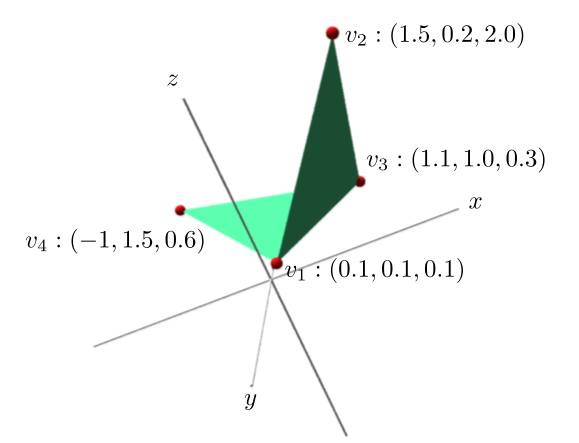It consists of 6 lines (the blank line is for the ease of human reading, and is ignored by the computer). The first 4 lines start with the letter v. They specify vertices. The three numbers following the v gives the $x$, $y$ and $z$ coordinates of that vertex. The vertices are numbered 1 onwards according to the order of listing.

Each line starting with an f specifies a (triangular) face. The three numbers are the indices of the three vertices.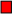## Visualising the end product

We want to write a dyad s that will take two inputs, the grid of $x$-values and the grid of $y$-values, and produce the .obj file for the surface of a function $F(x,y)$ (which we assume is already available). Here we are assuming that we shall always use the name F for the target function. Thus, a typical session to create the surface of $F(x,y)=\sin(x)\cos(y)$ could look like:
F=. 4 : '(sin x)*cos y'

x=. 10*(i: 100) %100

(x s x) fw <'test.obj' NB. Using the same grid for x and y

Here we have assumed that sin and cos are defined as usual, and that fw means "file write", ie,
fw =: 1!:2


## Doing the preparation

Let's chalk out the structure of the surface in terms of vertices and faces. We want all the faces to be triangular (such .obj files are the most portable). We can construct a Cartesian grid using the given $x$-grid and $y$-grid: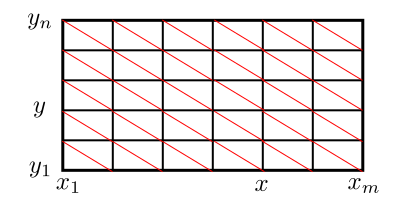We have drawn the red diagonals to split each cell into two triangular faces. Above each grid point we shall have a vertex on the surface. Thus, if there are $m$ values for $x$ and $n$ for $y,$ then the number of vertices would be $mn.$

## Writing the J code

### The vertices

For each grid point $(x,y)$ we need to produce a line like
v $x$ $y$ $F(x,y)$
In J this may be produced as
'v ' , ":number to string
x, y, (x F y)

We need to do this for each pair $(x,y).$ J makes this easy in the form
x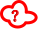/ y

What to place in the cloud? The answer is vx"0, where
vx=: 4 : 0
'v ', ": x, y, (x F y)
)

Notice that
x vx"0/ y

will produce a list of strings. We need to make a single string by joining them (after adding a newline character at the end).
newline=. , & LF
ver=. ,ravel
newline"1 x vx"0/ y

Well, that's all for the vertices.

### The faces

The faces are slightly more trickier to handle. If we have an $m\times n$ grid of vertices, then there are $(m-1)(n-1)$ cells, each being split into two triangles. We have numbered the vertices in a row-major fashion. So a typical cell will be like: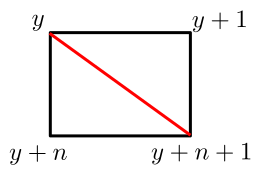This should produce two f lines:
f $y$ $(y+1)$ $(y+n+1)$
f $k$ $(y+n+1)$ $(y+n)$
It is easy to write a monad that will convert the input $y$ into a list of these two strings:
f=: 3 : 0
t1=. 'f ', ": y, (y+1), y+n+1
t2=. 'f ', ": y, (y+n+1), y+n
t1,: t2
)

Next we have to run this function over all the vertices. Wait, we have to actually run this over all the $(m-1)(n-1)$ cells. We are identifying each cell by its "top-left" vertex. So the function needs to run over all the blue vertices only in the following diagram: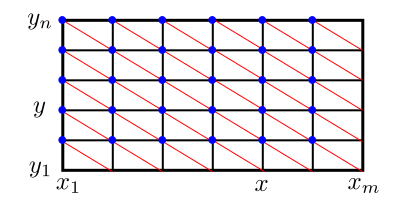Well, we need a list of all the blue vertices. We can make one from scratch. But let's use the pattern: all vertices except the last row and column. We start by making the whole grid:
mat=. >:1+
i. m, n

We needed to add 1 to start the counting from 1 (which is the norm with .obj format). Now tear off the last row:

}:curtail
mat

and then tear off the last entry of each of the remaining rows:
trim=. }:curtail
"1 }: mat

Now we may run our verb on it:
f"0 trim

This gives us an array of strings. We want to append a newline at the end of each, and join them:
fac=.,Ravel
newline"1 f"0 trim


### Putting the vertices and faces together

This is easy, just append them:
ver, fac

However, remember $J$'s habit of using underscore in place of minus. Well, .obj file format does not allow that. So we need to replace all the underscores by minuses:
'_-' charsub ver, fac


## The final code (raw version)

vx=: 4 : 0
'v ', ": x, y, (x F y)
)

fc=: 3 : 0
t1=.'f ', ": y, (y+1), y+ny+1
t2=.'f ', ": y, (y+ny+1), y+ny
t1,:t2
)

s=: 4 : 0
newline=. ,&LF
ver=: , newline"1 x vx"0/ y
nx=:  #x
ny=: #y
mat =: >:i. nx, ny
trim =: }:}:"1 mat
fac =: , newline"1 fc"0 trim
'_-' charsub ver,fac
)


## The final code (adverb version)

The above code is fine. But to use it you have to use the name F for the target function. This is irritating if you want to create .obj files for two functions in the same session. Notice that we had written a verb s that takes two inputs visibly (the $x$ and $y$ values) and one input invisibly (the target function). Thus, what we have written is alike a machine with three inputs, one of them being a verb. J calls such machines an adverb. Here a typical invocation would be
(x (myfun s) y) fw <'test.obj'

where myfun is the name of our target function. Earlier our s verb delegated its job to two verbs, vx for vertices and fc for the faces. We needed the target function for only the vertices. The faces are determined by the "topology" of the grid, and not on the exact positions of the vertices. So we need to pass the target function to vx also. This requires two modifications to our code: converting s and vx both to adverbs.
vx=: 1 : 0
:
'v ', ": x, y, (x u y)
)

fc=: 3 : 0
t1=.'f ', ": y, (y+1), y+ny+1
t2=.'f ', ": y, (y+ny+1), y+ny
t1,:t2
)

s=: 1 : 0
:
newline=. ,&LF
ver=: , newline"1 x (u vx)"0/ y
nx=:  #x
ny=: #y
mat =: >:i. nx, ny
trim =: }:}:"1 mat
fac =: , newline"1 fc"0 trim
'_-' charsub ver,fac
)